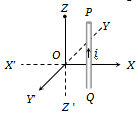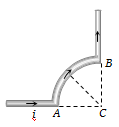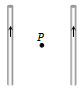A vertical wire kept in Z-X plane carries a current from Q to P (see figure). The magnetic field due to current-carrying wire will have the direction at the origin O along :(a) OX                                (c) OY

(b) OX'                               (d) OY'

Concept Questions :-

Biot-Savart law
High Yielding Test Series + Question Bank - NEET 2020

Difficulty Level:

The magnetic field at the centre of a coil of n turns, bent in the form of a square of side 2 l, carrying current i, is :

(a)  $\frac{\sqrt{2}{\mathrm{\mu }}_{0}\mathrm{ni}}{\mathrm{\pi l}}$                               (b)  $\frac{\sqrt{2}{\mathrm{\mu }}_{0}\mathrm{ni}}{2\mathrm{\pi l}}$

(c)  $\frac{\sqrt{2}{\mathrm{\mu }}_{0}\mathrm{ni}}{4\mathrm{\pi l}}$                                (d)  $\frac{2{\mathrm{\mu }}_{0}\mathrm{ni}}{\mathrm{\pi l}}$

Concept Questions :-

Magnetic field due to various cases
High Yielding Test Series + Question Bank - NEET 2020

Difficulty Level:

In a current carrying long solenoid, the field produced does not depend upon :

(a) Number of turns per unit length

(b) Current flowing

(d) All of the above three

Concept Questions :-

Ampere circuit law
High Yielding Test Series + Question Bank - NEET 2020

Difficulty Level:

A circular coil 'A'  has a radius R and the current flowing through it is I. Another circular coil ‘B’ has a radius 2R and if 2I is the current flowing through it, then the magnetic fields at the centre of the circular coil are in the ratio of (i.e. ${B}_{A}$ to ${B}_{B}$):

1. 4 : 1

2. 2 : 1

3. 3 : 1

4. 1 : 1

Concept Questions :-

Magnetic field due to various cases
High Yielding Test Series + Question Bank - NEET 2020

Difficulty Level:

A straight wire of diameter 0.5 mm carrying a current of 1 A is replaced by another wire of 1 mm diameter carrying the same current. The strength of the magnetic field far away is :

(a) Twice the earlier value

(b) Half of the earlier value

(c) Quarter of its earlier value

(d) Unchanged

Concept Questions :-

Magnetic field due to various cases
High Yielding Test Series + Question Bank - NEET 2020

Difficulty Level:

Which of the following gives the value of the magnetic field according to, Biot-Savart’s law :

(a)  $\frac{\mathrm{i}∆\mathrm{lsin}\left(\mathrm{\theta }\right)}{{\mathrm{r}}^{2}}$                               (b)  $\frac{{\mathrm{\mu }}_{0}}{4\mathrm{\pi }}\frac{\mathrm{i}∆\mathrm{lsin}\left(\mathrm{\theta }\right)}{\mathrm{r}}$

(c)  $\frac{{\mathrm{\mu }}_{0}}{4\mathrm{\pi }}\frac{\mathrm{i}∆\mathrm{lsin}\left(\mathrm{\theta }\right)}{{\mathrm{r}}^{2}}$                          (d)   $\frac{{\mathrm{\mu }}_{0}}{4\mathrm{\pi }}\mathrm{i}∆\mathrm{lsin}\left(\mathrm{\theta }\right)$

Concept Questions :-

Biot-Savart law

Difficulty Level:

A wire carrying current i is shaped as shown. Section AB is a quarter circle of radius r. The magnetic field is directed :(a) At an angle $\mathrm{\pi }/4$ to the plane of the paper

(b) Perpendicular to the plane of the paper and directed in to the paper

(c) Along the bisector of the angle ACB towards AB

(d) Along the bisector of the angle ACB away from AB

Concept Questions :-

Magnetic field due to various cases
High Yielding Test Series + Question Bank - NEET 2020

Difficulty Level:

Two long straight wires are set parallel to each other. Each carries a current i in the same direction and the separation between them is 2r. The intensity of the magnetic field midway between them is(a) ${\mu }_{0}i/r$                               (c) Zero

(b) $4{\mu }_{0i/r}$                              (d) ${\mu }_{0}i/4r$

Concept Questions :-

Magnetic field due to various cases
High Yielding Test Series + Question Bank - NEET 2020

Difficulty Level:

The ratio of the magnetic field at the centre of a current-carrying coil of the radius 'a' and at a distance ‘a’ from centre of the coil along the axis of the coil is:

1. $\frac{1}{\sqrt{2}}$                              2. $\sqrt{2}$

3. $\frac{1}{2\sqrt{2}}$                             4. $2\sqrt{2}$

Concept Questions :-

Magnetic field due to various cases
High Yielding Test Series + Question Bank - NEET 2020

Difficulty Level:

The earth’s magnetic field at a given point is $0.5×{10}^{-5}Wb-{m}^{-2}.$ This field is to be annulled by magnetic induction at the center of a circular conducting loop of radius 5.0cm. The current required to be flown in the loop is nearly :

(a) 0.2 A                   (b) 0.4A

(c) 4A                       (d) 40A

Concept Questions :-

Magnetic field due to various cases